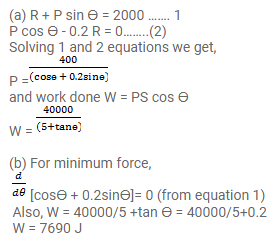# A box weighing 2000 N is to be slowly slid through

Question:

A box weighing $2000 \mathrm{~N}$ is to be slowly slid through $20 \mathrm{~m}$ on a straight track having friction coefficient $0.2$ with the box.

(a) Find the work done by the person pulling the box with a chain at an angle 9 with the horizontal.

(b) Find the work when the person has chosen a value of 0 which ensures him the minimum magnitude of the force.

Solution: